Note: This is an archvied version of our old webpage. Some links might be broken. The current one can be found here.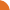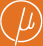Chair for Foundations of Software Reliability and Theoretical Computer Science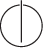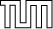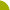Publications - Computing the Least Fixed Point of Positive Polynomial SystemsReference:

Javier Esparza, Stefan Kiefer, and Michael Luttenberger. Computing the least fixed point of positive polynomial systems. SIAM Journal on Computing, 39(6):2282–2335, 2010.

Abstract:

We consider equation systems of the form X_1 = f_1(X_1, ..., X_n), ..., X_n = f_n(X_1, ..., X_n) where f_1, ..., f_n are polynomials with positive real coefficients. In vector form we denote such an equation system by X = f(X) and call f a system of positive polynomials, short SPP. Equation systems of this kind appear naturally in the analysis of stochastic models like stochastic context-free grammars (with numerous applications to natural language processing and computational biology), probabilistic programs with procedures, web-surfing models with back buttons, and branching processes. The least nonnegative solution mu f of an SPP equation X = f(X) is of central interest for these models. Etessami and Yannakakis have suggested a particular version of Newton's method to approximate mu f.

We extend a result of Etessami and Yannakakis and show that Newton's method starting at 0 always converges to mu f. We obtain lower bounds on the convergence speed of the method. For so-called strongly connected SPPs we prove the existence of a threshold k_f such that for every i >= 0 the (k_f+i)-th iteration of Newton's method has at least i valid bits of mu f. The proof yields an explicit bound for k_f depending only on syntactic parameters of f. We further show that for arbitrary SPP equations Newton's method still converges linearly: there are k_f>=0 and alpha_f>0 such that for every i>=0 the (k_f+alpha_f i)-th iteration of Newton's method has at least i valid bits of mu f. The proof yields an explicit bound for alpha_f; the bound is exponential in the number of equations, but we also show that it is essentially optimal. Constructing a bound for k_f is still an open problem. Finally, we also provide a geometric interpretation of Newton's method for SPPs.

Suggested BibTeX entry:

@article{EKL10:SICOMP,
author = {Javier Esparza and Stefan Kiefer and Michael Luttenberger},
journal = {SIAM Journal on Computing},
number = {6},
pages = {2282--2335},
title = {Computing the Least Fixed Point of Positive Polynomial Systems},
volume = {39},
year = {2010}
}PDF (845 kB)Tech report version